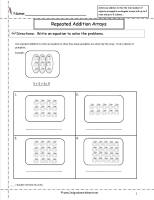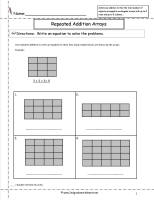﻿ CCSS 2.OA.4 Worksheets

# Common Core State Standard 2.OA.4

## Operations & Algebraic Thinking

### Work with equal groups of objects to gain foundations for multiplication.

4. Use addition to find the total number of objects arranged in rectangular arrays with up to 5 rows and up to 5 columns; write an equation to express the total as a sum of equal addends.

###Repeated Addition Arrays

Common Core State Standards: 2.OA.4
Operations & Algebraic Thinking
Work with equal groups of objects to gain foundations for multiplication.

Use addition to find the total number of objects arranged in rectangular arrays with up to 5 rows and up to 5 columns; write an equation to express the total as a sum of equal addends.

###Repeated Addition Arrays

Common Core State Standards: 2.OA.4
Operations & Algebraic Thinking
Work with equal groups of objects to gain foundations for multiplication.

Use addition to find the total number of objects arranged in rectangular arrays with up to 5 rows and up to 5 columns; write an equation to express the total as a sum of equal addends.

###Repeated Addition Arrays

Common Core State Standards: 2.OA.4
Operations & Algebraic Thinking
Work with equal groups of objects to gain foundations for multiplication.

Use addition to find the total number of objects arranged in rectangular arrays with up to 5 rows and up to 5 columns; write an equation to express the total as a sum of equal addends.